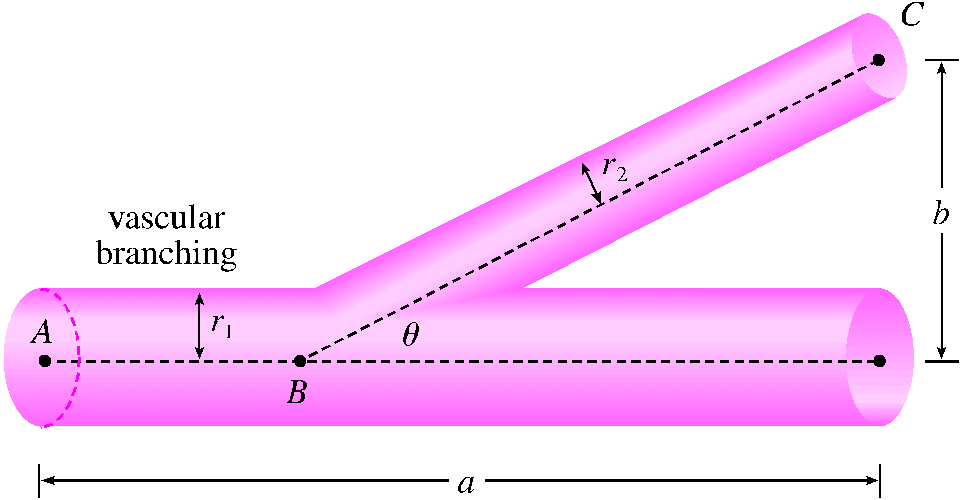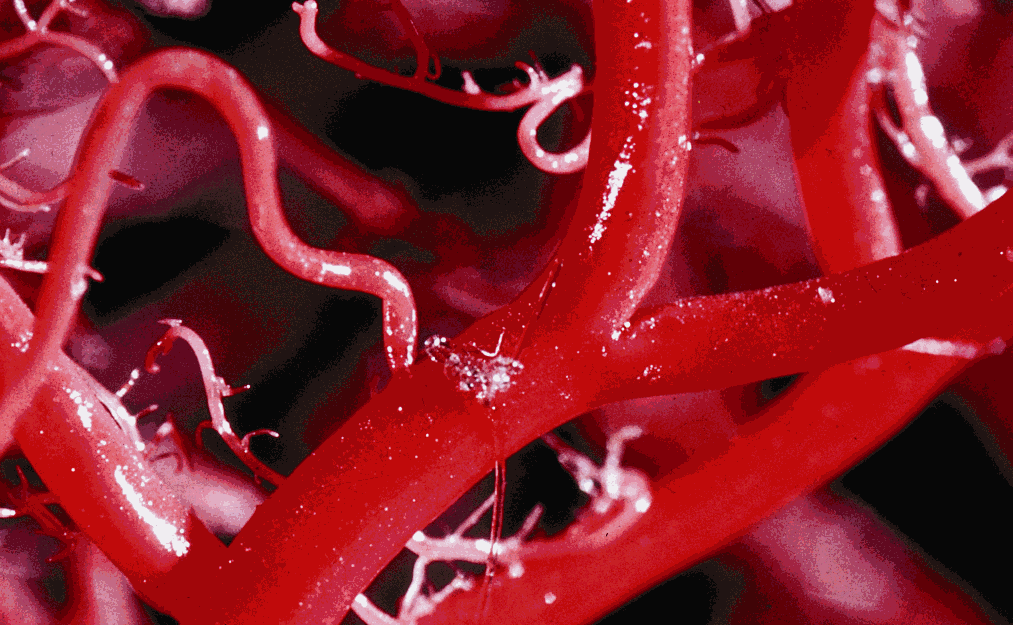The blood vascular system consists of blood vessels (arteries, arterioles, capillaries, and veins) that convey blood from the heart to the organs and back to the heart. This system should work so as to minimize the energy expended by the heart in pumping the blood. In particular, this energy is reduced when the resistance of the blood is lowered. One of Poiseuille's Laws gives the resistance $R$ of the blood as where $L$ is the length of the blood vessel, $r$ is the radius, and $C$ is a positive constant determined by the viscosity of the blood. The figure shows a main blood vessel with radius $r$ branching at an angle $\theta$ into a smaller vessel with radius $s$. (Note in your answers use $t$ instead of $\theta$ and $r$ and $s$ respectively instead of $r_1$ and $r_2$.)(a) Use Poiseuille's Law to find the total resistance of the blood along the path $ABC$ where $a$ and $b$ are the distances shown in the figure. (Your answer may depend on $a$, $b$, $C$, $r$, $s$, and $t$.)

$R$ =

(b) Find the value of $\cos \theta$ which minimizes the resistance. (Your answer may depend on $a$, $b$, $C$, $r$, and $s$.)

$\cos \theta$ =

(c) Find the optimal branching angle when the radius of the smaller blood vessel is $1/2$ the radius of the larger vessel.

$\theta$ =You can earn partial credit on this problem.